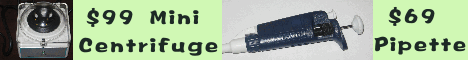The Electronic Protocol Book Table of contents BioToolKit 300 Download Trials An electronic protocol book with 500 protocols and 100 recipes. A great quick and practical reference for bench scientists as well as for new students.   Get A Copy A collection of tools frequently used by bench biomedical scientists, ranging from centrifugation force conversion, molecular weight, OD, recipe calculators, to clinical calculators. Include all Primo 3.4, Abie 3.0, Heatmap Viewer, MicroHelper, Godlist Manager, label printing, and grade book.   More info Estimate Sample size Sample size for two samples A pilot study found that the dissociation constant (Kd) for ligand A and B are 9.5 +/- 1.2 nM and 5.7 +/- 1.5 nM. How many samples do we need to show KdA - KdB > 3 nM at the 95% confidence level? We may use the estimator for testing m1 - m1 = D (one-sided, independent). Reasonable estimates of parameters are a = 0.05 (95% CI), b = 0.1, D = 3, s = 1.5. Required sample size will be 5, i.e., the binding assays should be repeated at least 5 times. For paired two samples, the differences should be calculated first, then treated as a one sample problem, i.e., testing for m (difference) = D. Sample size for one sample In a microarray experiment, we would like to find out how many duplicate arrays needed for reliably claiming a gene's transcription is at least 2-fold up/down compared to the control. Suppose we are using cDNA arrays and the data is in the log-scale (log2R/G). Using the estimator for testing m = D (two-sided) and the following parameters: a = 0.01 (99% CI), b = 0.1, D = log22 = 1, s = log21.5 = 0.4 (1.5 fold up/down), we find the required sample size is 3. Sample size for confidence interval A research would like to determine a mutant fruit fly's lifetime to the accuracy of +/- 5 days. On average flies live for 70 +/- 10 days. Using the sample size estimator for confidence intervals, he finds that he needs to measure the lifetime for at least 16 individual fruit files (a = 0.05, E = 5, s = 10). Sample size for Pearson correlation A researcher has found that gene A's activity is proportional to gene B's activity (ya ~ k yb ). To quantitate the effect she would like to determine the ratio to the relative accuracy of +/- 20%. Note k is proportional to the Pearson correlation r and the relative error in k is equivalent to the absolute error E in r. She used sample size estimator for Pearson correlation and found at 95% CI (a = 0.05), the required sample size is 68.What is a USB Flash Disk?